## research papersJOURNAL OFSYNCHROTRONRADIATION
ISSN: 1600-5775
Volume 6| Part 6| November 1999| Pages 1106-1114

## The use of a Hamamatsu X-ray image intensifier with a cooled CCD as a solution X-ray scattering detector

aStructural Biophysics Laboratory, RIKEN Harima Institute/SPring-8, SPring-8, 1-1-1 Kouto, Mikazuki, Sayo Hyogo 679-5148, Japan, bDivision of Biophysical Engineering, Graduate School of Engineering Science, Osaka University, Machikaneyama, Toyonaka 560-8531, Japan, and cExperimental Research Division, JASRI (Japan Synchrotron Radiation Research Institute), SPring-8, 1-1-1 Kouto, Mikazuki, Sayo, Hyogo 679-5198, Japan
*Correspondence e-mail: fujisawa@sp8sun.spring8.or.jp

(Received 8 March 1999; accepted 26 July 1999)

CCD detectors are now widely used in many synchrotron small-angle X-ray scattering beamlines. The use of an X-ray image intensifier with cooled CCD (XR-II + CCD) was studied, especially for use in synchrotron solution X-ray scattering. Two samples, polystyrene latex and apoferritin, were used. These two samples have fine structure in the solution scattering profile due to symmetry and narrow size distribution. The recorded scattering profile, in comparison with that obtained by a position-sensitive proportional counter (PSPC), showed that XR-II + CCD has a much smaller practical dynamic range (100:1) than that of a pixel well (7500:1). This limited dynamic range was overcome by placing various-size masks on the detection plane, thereby eliminating the high-intensity region. The images recorded with various masks were combined, and the reconstituted solution scattering profile was submitted to various analyses, including Guinier analysis, power-law analysis, size distribution analysis and calculation of radial density distributions. The results were the same as those obtained with the PSPC. This indicates that spatial distortion as well as shading, a decrease in sensitivity from the centre to the edge of the detecting region [Amemiya, Ito, Yagi, Asano, Wakabayashi, Ueki & Endo (1995). Rev. Sci. Instrum. 66, 2290–2294], have very little effect on the SAXS results. This paper presents a practical protocol for obtaining a reliable solution scattering profile given the limitations of XR-II + CCD for synchrotron solution X-ray scattering.

### 1. Introduction

Solution X-ray scattering is the scattering from particles in a solution, which includes polymers, lipids, micelles, proteins and nucleic acids. Because of its wide application, solution X-ray scattering therefore dominates the field of small-angle scattering. In order to clarify the problems in the use of a new detector, the characteristics of solution X-ray scattering are as follows.

(i) High intensity in the inner scattering region, especially around the beam stop, with a gradual decay in intensity (1/100–1/10000) toward the edge.

(ii) A weak signal in the region near the edge comparable with that of natural radiation.

(iii) Stability in the time scale (days or hours) in order to ensure identical conditions between sample and buffer profiles.

(iv) Uniformity in the detection area, since solution scattering analysis often uses the tangent to the intensity rather than the position of a peak.

(v) High resolution (∼200 µm) in the centre area, while near the edge low resolution (<1 mm) is sufficient because no sharp peaks are seen in the profile.

(vi) Capability of performing time-resolved experiments.

In the early days of solution scattering the detector was a scintillation counter, and this was later replaced by a position-sensitive proportional counter (PSPC). The successive derivatives of PSPCs, such as the quadrant detector and two-dimensional PSPC, used for solution scattering were all gas detectors (Gabriel, 1977; Fowler et al., 1983; Boulin et al., 1982). The integration-type detector represented by an imaging plate (IP) has not been used for quantitative measurements. The main problem with using an IP for solution scattering is that time-resolved experiments cannot be conducted. Furthermore, there is no systematic evaluation for solution X-ray scattering. One of the problems is the time-dependent feature of phosphorus decay, which makes it difficult to satisfy the third requirement in the above list.

The gas-type detector represented by the PSPC has been widely used in the second-generation synchrotron sources because it has long given satisfactory results. However, the modern synchrotron facilities with insertion devices such as wigglers or undulators changed the situation: standard PSPCs cannot tolerate the intense scattering from the sample. Besides, the CCD detector is more efficient than the standard PSPC system, which reduces both collection time and radiation damage on protein solutions. As a result many SAXS beamlines at second- or third-generation sources have started to employ the CCD-based detector as the main detector (Amenitsch et al., 1999; Bras et al., 1999; Fujisawa et al., 1997; Narayanan et al., 1999; Seifert et al., 1999). There are growing interests from SAXS users, especially solution scattering synchrotron users, who want to know how the familiar results of second-generation sources with the standard PSPC differ from those of third-generation sources with CCDs.

XR-II + CCD, developed by Amemiya's group (Amemiya et al., 1995), has two major merits: (a) a large dynamic range, (b) capability of performing time-resolved experiments. The drawbacks, on the other hand, are spatial distortion and shading effect, i.e. loss of detection efficiency especially in the region near the edge. These features are common to CCD-based detectors. Many studies on the application of XR-II + CCD to diffraction studies have been carried out, and a calibration program has been developed (Hammersley et al., 1994; Ito, 1998). On the other hand, there have been few studies on the application of this detector to solution scattering. The results of detector correction can be seen directly in the image in the case of diffraction, while solution scattering requires further processing and the detector correction is only apparent in the final stage of analysis. The CCD-based detector apparently compromises extra difficulties compared with standard PSPCs. However, there are no alternative detectors available for third-generation sources. In this paper we describe how to use the XR-II + CCD properly for the use of solution scattering, and the effects on their final results.

### 2. Materials and methods

#### 2.1. Detector

The detector is composed of (i) an X-ray image intensifier, which has a beryllium entrance window; (ii) an optical lens; (iii) a CCD used as an image sensor; and (iv) a data-acquisition system. The X-ray image intensifier (Hamamatsu, V5445P-MOD) has an aperture of 150 mm diameter with a 1 mm-thick beryllium window. Incident X-rays are converted into visible photons by the phosphor (CsI:Na) at the beryllium window, and they are then converted into photoelectrons by a photocathode. An output phosphor [Gd2O2S:Tb (P43), 13 mm-diameter] is used to convert photoelectrons into intensified visible light. Optical coupling between the image intensifier and the image sensor is performed by an optical lens. An iris (hereafter called aperture) of the CCD regulates the amount of light in the optical coupling. Two CCD sensors are used: one (Hamamatsu, C4880-80-14A) for time-resolved measurements (hereafter called CCDfast') and the other (Hamamatsu, C4880-10-14A) for static measurements (hereafter called CCDslow'). The signal from CCDslow is digitized by a 14-bit A/D converter and the signal from CCDfast is digitized by a 14-bit or 10-bit A/­D converter in static and real modes (28 Hz), respectively (Amemiya et al., 1995).

#### 2.2. Sample preparations

Polystyrene latex (PS) was purchased from Sekisui Chemical Co. PS is very stable against X-ray radiation and has a spherical structure. The mean diameter of N-100 (Sekisui Chemical Co.) is 1200 Å with 2.5% standard deviation. The solid content of PS was measured by weight after evaporation of water. In order to enhance contrast as well as reduce the fluctuation contribution to total scattering, solid PS was dissolved in glycerol. The final concentrations of latex and glycerol were 5.1 wt% and 52.1 wt%, respectively. Apoferritin was prepared from horse spleen ferritin (Sigma) by stepwise dialysis against 1 to 0% thioglycollic acids (TGC) in 0.1 M acetic acid/NaOH, 0.1 M NaCl, pH 5.5. The ferritin solution (10 mg ml−1) was dialyzed first against 1% TGC overnight and then repeatedly by 0.1% TGC containing 1 mM 2,2′-bipyridyl until the pink colour of the solution disappeared. The solution was then dialyzed against 0% TGC, followed by a change of dialysis buffer to 0.1 M HEPES, pH 7.0. The apoferritin concentration was determined from its absorption at 280 nm (e = 19500 M−1 cm−1 per subunit; Heusterpreute & Crichton, 1981).

#### 2.3. Solution scattering measurement by XR-II + CCD

Measurement of the solution scattering pattern was performed at RIKEN structural biology beamline I (BL45XU). A detailed description of the SAXS optics will be presented elsewhere (Fujisawa et al., 1999). The half size of the focus at BL45XU is 0.2 mm (vertical) × 0.4 mm (horizontal). The camera length was 2.2 m for PS, and 0.5 m and 1.8 m for apoferritin. The aperture of the CCD was determined so as to set the maximum charge of one pixel to less than 40000 e, which is ∼60–70% of a pixel well. Incident intensity was scaled by an ionization chamber current. A dark image was collected after each data collection with the same collection time but without X-ray irradiation, by which the transfer noise from the CCD camera to the interface board as well as that from the after-glow of the phosphor were recorded. Typical output of a dark image is within 200 A/D counts, most of which are offset counts for an A/D converter. The X-ray wavelength was 1.0 Å.

#### 2.4. Solution scattering measurement by PSPC

The reference data of XR-II + CCD were recorded by a SAXS camera at BL-10C, Photon Factory, Tsukuba (Ueki et al., 1985). The effective length of the PSPC was 200 mm with a delay line of 400 nS. The signal from the PSPC was converted to time–distance analogue information by a time-to-digital converter, then the time difference was converted to digital information of the position by a time-to-digital converter. The data were stored in histogram memory. The camera length was 200 cm and the X-ray wavelength was 1.488 Å.

A direct comparison of PSPC and XR-II + CCD was made at BL45XU at a wavelength of 1.0 Å. The effective length of the PSPC was 50 mm. The process of the output signal was basically the same as described above. The small area of the CCD image, which was identical to the detection area of the PSPC, was used for direct comparison.

#### 2.5. Preliminary data processing of the solution scattering pattern

Subtraction of the buffer image was performed by using the following equation,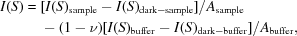where S = 2 sinθ/λ, 2θ is the scattering angle and λ is the wavelength. I(S), I(S)sample, I(S)buffer, I(S)dark-sample and I(S)dark-buffer are the circular-averaged intensity of subtracted intensity, intensity of the sample, intensity of the buffer, intensity of dark collection after the sample, and intensity of dark collection of the buffer, respectively. ν is the volume fraction of the solute and Asample and Abuffer are scaling factors of each intensity. The subtraction procedure was performed by using the Fortran program IISGNAPR (Fujisawa et al., 1997).

### 3. Results and discussion

#### 3.1. Effects of spatial distortion and shading by image intensifier curvature

The effects of spatial distortion and shading are discussed in this section. The distortion correction program is based on the difference in position and integrated intensity of spots of a grid-patterned mask between XR-II + CCD and IP. The distortion is approximated as a three-dimensional function, such as a cubic spline function, by which the redistribution of intensity and the position of each pixel was performed (Hammersley et al., 1994). When a direct beam is radiated into the centre of the detection area, the distortion of XR-II + CCD at a given radial pixel is independent of the azimuthal position of XR-II + CCD and only a function of the pixel distance from the centre in Fig. 1. The correction factor for distortion and shading was approximated by a polynomial function by least-squares fitting. When the correction curves of CCDslow and CCDfast were scaled by pixel size, they coincided. There are several possible reasons for these effects; for example, the beryllium window curvature, inhomogeneity of XR-II, and optical lens response. These effects are also dependent on sample-to-detector distance, which affects the incident angle of the X-rays on the detection plane.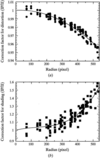Figure 1 Circular averaged correction factor for shading and deviation functions from CCDslow. The grid-patterned mask (10 mm × 10 mm) has 173 holes of diameter 2 mm. Water scattering was used. The sample-to-camera distance was 2.2 m. The correction factor, Y, is defined so that I(X)corrected = Y × I(X)apparent, where I(X)apparent and I(X)corrected are apparent and corrected circular averaged intensities at the radial pixel X. Y for each grid points were plotted as circles and squares for distortion and shading, respectively. The correction factors of distortion and shading were approximated by quadratic and bimodal equations, respectively (solid lines): Y = 1 + 8.82 × 10−6X − 1.71 × 10−7X2 and Y = 1 + 4.87 × 10−4X − 2.50 × 10−6X2 + 6.13 × 10−9X3 + 5.08 × 10−13X4 for deviation and shading, respectively.

#### 3.2. Direct comparison between standard PSPC and XR-II + CCD

The performance of XR-II + CCD was shown in solution scatterings from standard proteins with various molecular weights (Fig. 2). The scattering curves are identical although the quality of data with XR-II + CCD was superior to that of PSPC. The quality of PSPC data will be improved when Xe gas is used instead of Ar. Both data were identical; however, it should be noted that the incident beam should be attenuated (by ∼1/10) in order to keep the linearity of the PSPC.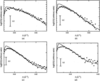Figure 2 Direct comparison of PSPC with XR-II + CCD. The solution scattering patterns from various protein solutions with both XR-II + CCD (solid lines) and PSPC (dotted lines) are shown. (a) Myoglobin (MW = 17.8 kDa). (b) Chicken egg albumin (MW = 45 kDa). (c) Bovine serum albumin (MW = 68 kDa). (d) Aldolase (MW = 158 kDa). All are dispersed in 10 mM HEPES (pH 7.0). Collection time was 10 s. For PSPC, Ar + CH4 gas was used. The incident beam was attenuated so that the rate of PSPC lies below 10 kcps in which the linearity was assured. The receiving slit before PSPC was 1 mm. The CCD area used for comparison was 0.9 mm × 50 mm. The data increment of PSPC was adjusted with a pixel size of XR-II + CCD. The small decrease in PSPC near the beam stop is due to optics alignment.

#### 3.3. Effect on the determination of radius of gyration

Based on these calibration features, the detector effect on the radius of gyration, Rg, is discussed. Rg determination by Guinier plot {ln[I(S)] versus S2 plot} uses the following formula,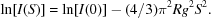The above formula indicates the following. (i) In the case of the shading effect of XR-II + CCD, the detected I(S) becomes smaller than the ideal decrease in I(S) as S increases, and therefore the tangent of the Guinier plot becomes steeper and, consequently, Rg becomes larger. (ii) On the other hand, the distortion effect of XR-II + CCD enlarges the horizontal direction in the Guinier plot, which reduces the tangent, i.e. Rg. The more pixels used, the larger the error in the determination of Rg. When two detector effects are compared, the distortion effect is negligible, since the Guinier plot uses the inner part of the detection area. The limit of the number of pixels for the determination of Rg is therefore determined by the shading effect which is the most important one. For example, Rg from CCDslow without correction lies within 1% error when 55 pixels from the centre of the detection plane were used. The determination of Rg was normally performed in the S region where 2πS [Å−1] Rg [Å] < 1.3. One pixel corresponds to (pixel size)/L, and therefore the camera length used for a given Rg [Å] should be less than L [mm] < Rg [Å] × 2π × 55 × (pixel size) [mm]/1.3, i.e. L [mm] < 40Rg [Å] for CCDslow. This equation is valid for CCDslow. In conclusion, the circular average of XR-II + CCD data can be used for Rg determination without a shading and deviation correction, if an appropriate camera length is chosen.

#### 3.4. The dynamic range of XR-II + CCD for an actual scattering image

The dynamic range of a detector is obtained by measuring the integrated intensities of small spots while changing the incident beam intensity. The dynamic range of XR-II + CCD is ∼6000:1 (Amemiya et al., 1995). The practical solution scattering image, however, is continuous and therefore may introduce another problem for the dynamic range; the intensity of one spot may influence a remote area, while the dynamic range of the spot is assured. We used polystyrene latex in reverse contrast media as a standard sample for evaluation, since PS is a solid sphere and its scattering can be accurately approximated by the form factor of a solid sphere. In comparison with the PSPC, XR-II + CCD deviated in the S region where the scattering is less than 1/100 of the highest intensity (Fig. 3a). When the high-intensity regions were masked, XR-II + CCD recorded the same solution scattering as that recorded by the PSPC (Fig. 3b). This indicates that the practical dynamic range is 100:1, far below the pixel depth. The situation is much worse when CCDfast is used; the actual dynamic range was 40:1 (data not shown here). The most important point is that this effect cannot be corrected geometrically. One possible explanation for this effect is the contrast decrease in XR-II, for example, stray light in output window (Ito, 1998). Another possible explanation is that it comes from the lens optics. High intensity causes interference in a lens like a `cataract'.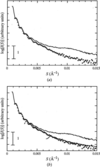Figure 3 Solution scattering pattern from polystyrene latex. The weight concentration of polystyrene latex was 5.1% dispersed in 52.1 wt% glycerol. (a) Here, the patterns start to deviate below 1/100 of the highest I(S). The solid line was obtained with an aperture of 10, 350 ms of collection time, and no mask. The open circles were obtained by using a PSPC. (b) Here, it is shown that the above effect can be compensated by masks. The broken line was obtained with an aperture of 7, 10 s of collection time and 15 mm-diameter mask. The chain-dotted line was obtained with an aperture of 4, 12 s of collection time and 30 mm-diameter mask.

When the dynamic range is within limits, circular integration of the detected A/D counts greatly reduces the apparent signal-to-noise ratio. However, it should be noted that a sufficient quantum of A/D counts for one pixel is necessary to avoid digitization errors. A/D counts less than 2 after background subtraction often resulted in deviations from the ideal solution scattering profile.

#### 3.5. Power law analysis

Here we deal with power law analysis, which uses a wider scattering region than that of the Guinier plot. Introducing various masks onto the detection plane in order to maintain a dynamic range of 1:100 as well as collecting sufficient A/D counts gave the reconstituted scattering curve shown in Fig. 4. The scattering intensity of a solid sphere should theoretically follow the S−4 law, and the PS scattering from the PSPC as well as the reconstituted curve from XR-II + CCD does follow this law. The upper limit of fitting, 120 pixels from the centre of the detector, can be considered as the limit of this analysis without detector corrections.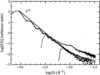Figure 4 Power-law tendency of the solution scattering pattern from polystyrene latex. Polystyrene latex is accurately approximated by a solid sphere with a smooth surface, which gives a S−4 decay. Symbols are the same as those in Fig. 3. The solution scattering profile obtained by XR-II + CCD follows the S−4 law.

#### 3.6. Determination of size distribution

In the following section the effects of shading and distortion on the final results of solution scattering analysis, which includes inverse Fourier transformation, are discussed. The mathematical transformation often results in an exaggeration of detector distortions and is more conspicuous in the final result than in the analysis in reciprocal space. One of the most sensitive methods is the regularization method for particle size distribution N(D), which estimates the volume-weighted size distribution of particles with diameter D. This is performed by solving the following equations,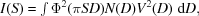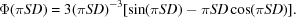In these equations function V(D) relates D to the particle volume. The solution of the equations is usually not stable and is greatly affected by the scattering curve itself. The volume-weighted size distribution N(D)V2(D) was calculated by using the software package SIZES based on the regularization method (Jemian, 1995). The final fit of the experimental data as well as the volume-weighted size distribution N(D)V2(D) are shown in Fig. 5. The peak diameter was 1250 Å and the half width of the distribution was 3.8%, which are consistent with those characterized by light scattering. It should be noted that the scattering curves from XR-II + CCD are not corrected for distortion and shading effects. Detector correction for both shading and distortion has very little effect on the final data.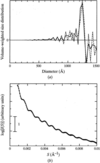Figure 5 Size distribution obtained from a reconstituted SAXS profile by XR-II + CCD. (a) Size distributions calculated from SAXS profiles without correction for detector distortion (solid line) and with correction (broken line). Within this region it is very difficult to see the difference between these two. The peaks of the size distributions are at 1250 Å with a half width of 43 Å and at 1250 Å with a half width of 48 Å, with and without detector correction. (b) The fitting of (a) in scattering intensity. Solid circles and open circles are data points with and without detector correction, respectively. The solid line and broken lines are fitted scattering intensity with and without detector correction, respectively.

#### 3.7. Box refinement analysis on apoferritin

If a particle has spherical symmetry its radial electron density can be determined by using the box refinement method. This method is also greatly affected by the shape of the solution scattering profile and therefore could be a good test for detector usage. Apoferritin is a protein complex consisting of a hollow spherical shell made of 24 protein chains arranged in a 432-point symmetry (Smith et al., 1989). The protein complex incorporates Fe+++ ions inside its shell and transports them across the cell membrane. Scattering from a spherical shell has a steep trough, which is easily smeared by detector effects. Fig. 6shows the detector effect on the solution scattering profile of apoferritin. By applying various masks, smearing of the first trough was overcome, but there are still discrepancies for the second and third troughs. In dealing with a protein system the possibility of radiation damage cannot be ruled out. The smearing of troughs by changing different sample preparations was observed even with a PSPC, although they were much smaller than these with the XR-II + CCD. The limit to the dynamic range could not be compensated by using masks in the case of a solution scattering profile with steep troughs (1/100 of peak maximum).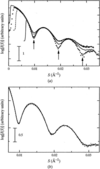Figure 6 The smearing effect observed in apoferritin. (a) Scattering profile of apoferitin 11 mg ml−1 solution. No mask (solid line), 15 mm-diameter mask (dotted line), 30 mm-diameter mask (chain-dotted line), and measured by PSPC (open circle). The valleys of the scattering are shown by arrows. (b) The charge effect characterized by changing collection time: 3 s (solid line), 2 s (broken line) and 1 s (dotted line). All profiles were taken with an aperture of 4.

If the smearing of troughs cannot be avoided, the next question is whether or not smearing affects the final results. For particles with spherical symmetry it is theoretically possible to calculate the radial electron density ρ(r) from the scattering intensity since, as a consequence of symmetry, no information is lost in the process of spherical averaging. The scattering amplitude A(S) = ±[I(S)]1/2 relates to ρ(r) by the following equation,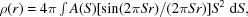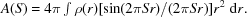If the signs of the scattering amplitude are determined, ρ(r) can be restored. This solution to the sign (phase) problems is obtained under the condition where ρ(r) is finite, i.e. Router > r > Rinner, ρ(r) = 0, where Router and Rinner are values of the outer and inner radii of the shell, respectively. First, the phase is constructed by an arbitrary value of ρ(r). Then using that phase, ρ*(r), ρ(r) of the next iteration, is calculated but it is not finite. By imposing the condition Router > r > Rinner, ρ*(r) = 0, ρ(r) of the next iteration is constructed. Based on that ρ(r), the phases are recalculated and used for producing ρ(r) at the next iteration. The continuation of the iteration converges to a true ρ(r) (Svergun et al., 1984). Since this analysis is affected not only by the peak position but also by the slope of each peak, it could be a good detector test.

Fig. 7shows radial electron density distributions of apoferritin taken from PSPC and XR-II + CCD and their fitting to experimental data. In the case of a solid spherical shell, ρ(r) becomes a step function, ρ(r) ≠ 0 in Router > r > Rinner. Since the X-ray crystallographic structure of apoferritin complex has been solved (Hempstead et al., 1997), from the atomic coordinates one can also calculate the radial electron density distribution. The difference between PSPC and XR-II + CCD was very small compared with the resolution from the algorithm itself: the peak position of radial electron density and Router and Rinner basically coincide with each other. The value of ρ(r) from XR-II + CCD is similar to that from crystal coordinates.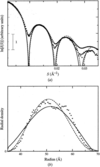Figure 7 Calculation of the radial density distribution from apoferritin. Fitting result of radial density distribution (a) and its corresponding result (b). Solid circles are solution scattering from XR-II + CCD, open squares are those from PSPC. The broken and solid lines are the fitting of XR-II + CCD and PSPC data in the range S < 0.033 Å−1, respectively. (b) Corresponding results of fitting are shown. Symbols are the same as in (a).

Fig. 8demonstrates the power in the detection efficiency of XR-II + CCD. By using two-dimensional data, a fine structure in a medium-angle region can easily be obtained. The ripples are supposed to derive from the form factor of the shell.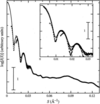Figure 8 Reconstitution of the solution scattering pattern from apoferritin. The solid line was recorded with a 220 cm camera, with an aperture of 10, 3 s data collection and no mask. The range S < 0.008 Å−1 was used. The broken line was recorded with a 220 cm camera with an aperture of 4, 4 s data collection and 30 mm-diameter mask. This curve was used with S < 0.0165 Å−1. Open circles were recorded with a 50 cm camera using an aperture of 7, 100 s (10 s × 10 cycle), and 15 mm-diameter mask. The same curves are magnified in the insert. Crosses were recorded by a PSPC in a 2400 s exposure. The protein concentration was 11 mg ml−1.

### 4. Conclusions

Table 1summarizes the comparison among standard SAXS detectors. XR-II + CCD has detector distortion and shading, as well as a low practical dynamic range. It is not an appropriate detector for standard X-ray generators. Despite these drawbacks, this detector has very attractive features; high efficiency, short readout time, capability of time-resolved experiment (>1 ms), tolerance of high dose and easy maintenance. These advantages are essential to modern synchrotron beamlines, therefore this detector will be used in various synchrotron facilities for the time being. This limit of dynamic range can be overcome by using appropriate masks. The practical protocol for obtaining reliable solution scattering is as follows.

 Table 1Comparison of standard SAXS detectors
Delay line PSPC XR-II + CCD Imaging plate
Pixel size (mm) 0.2–1 0.15–0.25 0.025–0.2
Number of pixels 128–1024 1024 × 1024 2000 × 4000
Maximum rate (s mm−2) 104 5 × 107 106
Maximum total rate (counts s−1) 3 × 105
Efficiency 0.15–0.3 0.6–0.8 0.4
Counts per photon 1 0.2–20 1–2
Effective dynamic range 104 102 103–104
Readout time per frame 0.01 ms 33 ms–2 s 12–120 s
†Amemiya et al. (1995).

(i) Set the time and aperture so that the maximum intensity becomes 60 or 70% of the pixel depth.

(ii) Record the dark image after every sample and buffer measurement.

(iii) The net signal should be large enough to avoid digitization error. Parasitic scattering should therefore be minimized so as to maximize the net count in the limited data-collection time.

(iv) Practical dynamic ranges for CCDfast and CCDslow are 40:1 and 100:1, respectively. The high-intensity region should be masked.

(v) In the case of Guinier analysis, a shorter camera length is better; for example, L [mm] < 40Rg [Å], if there are no distortion and shading effects.

As long as the centre of the detection plane is used for analysis, the following samples can be measured without correction of distortion and shading:

(i) Protein solutions (Guinier analysis, distance distribution function and Kratky plot);

(ii) Polymer solutions (Fractal analysis);

(iii) Protein with high symmetry, such as virus shell protein complex.

In the case of a protein solution, the peak height of the Kratky plot is only relevant, for which the centre of the detector is used. When the slope or oscillation of the Kratky plot is relevant, it uses the edge region and the image needs special care. The calculation of the distance distribution function is more stable than that of the radial electron density distribution. When the values of Rg and I(S) are identical to those of the PSPC, most indirect methods give the same results.

### Acknowledgements

Dr Hidenori Toyokawa kindly helped with PSPC measurements and analysis at SPring-8. The authors thank Professor Yoshiyuki Amemiya and Dr Kazuki Ito for helpful discussions and comments in contrast effect of XR-II. Dr Tatzuo Ueki directed the beamline construction (BL45XU) at SPring-8. Dr Katsumi Kobayashi at the Photon Factory played a key role in maintenance of BL-10C at the Photon Factory. The synchrotron radiation experiments were performed at SPring-8 with the approval of the Japan Synchrotron Radiation Research Institute (JASRI) (proposal No. 1998A0102-CL-np). Part of this work (experiments at the Photon Factory) has been performed under the approval of the Photon Factory Advisory Committee (proposal No. 97G123). This work was supported by both the special coordination funds for promoting science and technology and SPring-8 Joint Research Promotion Scheme of Japan Science and Technology Corporation.

### ReferencesJOURNAL OFSYNCHROTRONRADIATION